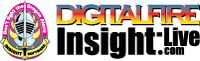•The secret to cool bodies and glazes is a lot of testing.
•The secret to know what to test is material and chemistry knowledge.
•The secret to learning from testing is documentation.
•The place to test, do the chemistry and document is an account at https://insight-live.com
•The place to get the knowledge is https://digitalfire.com

Sign-up at https://insight-live.com today.

# Hallam Fireclay

 Oxide Analysis Formula CaO 0.30% 0.025 MgO 0.15% 0.018 K2O 0.40% 0.020 Na2O 0.80% 0.061 TiO2 0.87% 0.051 Al2O3 21.60% 1.000 SiO2 66.50% 5.225 Fe2O3 1.72% 0.051 H2O 7.00 Oxide Weight 436.05 Formula Weight 468.87

## XML for Import into INSIGHT

<?xml version="1.0" encoding="UTF-8"?> <material name="Hallam Fireclay" descrip="" searchkey="" loi="0.00" casnumber=""> <oxides> <oxide symbol="CaO" name="Calcium Oxide, Calcia" status="" percent="0.300" tolerance=""/> <oxide symbol="MgO" name="Magnesium Oxide, Magnesia" status="" percent="0.150" tolerance=""/> <oxide symbol="K2O" name="Potassium Oxide" status="" percent="0.400" tolerance=""/> <oxide symbol="Na2O" name="Sodium Oxide, Soda" status="" percent="0.800" tolerance=""/> <oxide symbol="TiO2" name="Titanium Dioxide, Titania" status="" percent="0.870" tolerance=""/> <oxide symbol="Al2O3" name="Aluminum Oxide, Alumina" status="" percent="21.600" tolerance=""/> <oxide symbol="SiO2" name="Silicon Dioxide, Silica" status="" percent="66.500" tolerance=""/> <oxide symbol="Fe2O3" name="Iron Oxide, Ferric Oxide" status="" percent="1.720" tolerance=""/> </oxides> <volatiles> <volatile symbol="H2O" name="Water" percent="7.000" tolerance=""/> </volatiles> </material>